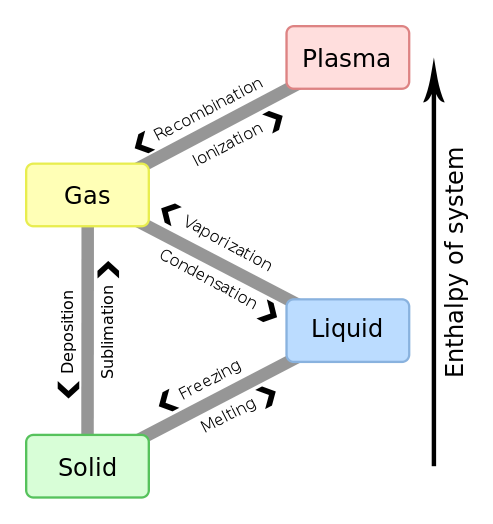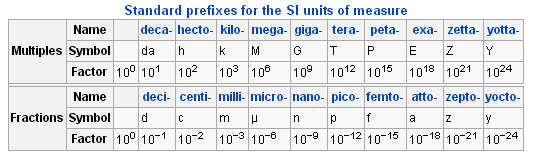Substances that Exist as Gases

Substances that exist in the gas phase exhibit negligible intermolecular forces.

Key Takeaways

Key Points

• A gas is one of the three classical states of matter (the others being liquid and solid ).
• Under standard temperature and pressure (STP, or 1 atm and 273 K), a substance which exists as a gas is called a pure gas.
• A pure gas may be made up of individual atoms (e.g. a noble gas or atomic gas like neon), elemental molecules made from one type of atom (e.g. oxygen ), or compound molecules made from a variety of atoms (e.g. carbon dioxide).
• If the boiling point of a substance is below 273 K, then the substance to be in gas form at STP.

Key Terms

• absolute zero: the coldest possible temperature, zero on the Kelvin scale, or approximately −273.15 °C, −459.67 °F; total absence of heat; temperature at which motion of all molecules ceases

Gas as a State of Matter

Gas is one of the three classical states of matter (the others being liquid and solid). Near absolute zero, a substance exists as a solid. As heat is added to this substance it melts into a liquid at its melting point, boils into a gas at its boiling point, and if heated high enough would enter a plasma state in which the electrons are so energized that they leave their parent atoms from within the gas.States of matter: Matter transitions between three classical states of matter (gas, solid, and liquid) and a fourth state of matter. Note that the enthalpy of a system is the heat content of a system at constant pressure. The process of a solid converting to a liquid is known as “melting”; liquid to a gas is “vaporization”; and gas back to a solid is “deposition.” These same processes in the reverse direction are “freezing”; “condensation”; and “sublimation.”

Kinetic Theory of Matter

Why does matter exist in three different states? The Kinetic Theory of Matter provides a basic overview:

• Matter is made up of constantly-moving particles.
• All particles have energy, but the energy varies depending on whether the substance is a solid, liquid, or gas; solid particles have the least amount of energy and gas particles the most.
• The temperature of a substance is a measure of the average kinetic energy of the particles.
• A change in phase may occur when the energy of the particles changes.
• There are spaces between the particles of matter.
• There are attractive forces between particles and these become stronger as the particles move closer together. These attractive forces are known as intermolecular forces. An ideal gas is assumed to experience no intermolecular forces whatsoever, due to the fact that the particles of an ideal gas are moving so quickly, and are so far apart from one another, that they do not interact at all.
• Gases behave most ideally at high temperatures and low pressures. This is because under these conditions, intermolecular forces will be minimized.

Interactive: Molecular View of a Gas: Explore the structure of a gas at the molecular level. Note that unlike solids, gases do not follow a rigidly patterned structure; at a microscopic level, gases are always moving and rearranging themselves.

We discussed the basic states of matter in which a substance can be interconverted depending on conditions. Under standard conditions (1 atm, 273 K), a substance which exists as a gas is called a pure gas and (disregarding any substance-specific intermolecular forces or particle volume that could alter this value) has a volume of 22.4 L per mole. A pure gas may be made up of individual atoms (e.g. a noble gas or atomic gas like neon), elemental molecules made from one type of atom (e.g. oxygen), or compound molecules made from a variety of atoms (e.g. carbon dioxide). At STP, if the boiling point of a given substance is below 273 K then you would expect that substance to be in gas form.

Interactive: What is Pressure?: Gases have no definite shape or volume. When they are constrained to a container, we can measure the pressure they exert on the container walls. The model shows the inside (yellow atoms) and outside (green atoms) of a balloon. The teal barrier represents the wall of the balloon. Add atoms to the balloon and watch what happens. Because of the increased pressure, the volume increases!

Elemental Gases

The group VIII elements (helium (He), neon (Ne), argon (Ar), krypton (Kr), xenon (Xe) and radon (Rn)) exist as monatomic gases at standard temperature and pressure (STP) and are called the noble gases. The only other elements which exist as gases at STP  are hydrogen (H2), nitrogen (N2) and oxygen (O2), plus the two halogens, fluorine (F2) and chlorine (Cl2). These gases, when grouped together with the monatomic noble gases are called “elemental gases. ”Periodic Table of Elements: Nobles Gases (Group VIII) exist as gases at STP.

SI Units of Pressure

The SI unit of pressure is the pascal (Pa), which is equal to one Newton per meter squared (N/m2).

Learning Objectives

Recognize the relationship between derived and base SI units

Key Takeaways

Key Points

• The International System of Units (SI) is the basis of the modern metric system. All SI units can be derived from the seven fundamental SI units.
• Ranges of specific units are indicated by positive or negative multiples of powers of ten (e.g. 102, 10-2, etc.).
• Pressure —the effect of a force applied to a surface—is a derived unit, obtained from combining base units.
• The unit of pressure in the SI system is the pascal (Pa), defined as a force of one Newton per square meter.
• The conversion between atm, Pa, and torr is as follows: 1 atm = 101325 Pa = 760 torr.
• A standardized prefix system indicates fractions and multiples of metric units (e.g. milli-, mega-).

Key Terms

• International System of Units: the basis of the metric system; SI, from the French Système international d’unités; metric measurements derive from seven base units and multiples of ten
• Newton: in the International System of Units, the derived unit of force; the force required to accelerate a mass of one kilogram by one meter per second per second; symbol: N
• pascal: in the International System of Units, the derived unit of pressure and stress, equal to one newton per square meter; symbol: Pa
• Pressure: The amount of force applied over a given area divided by the size of the area.
• barometer: an instrument for measuring atmospheric pressure

SI Units

The International System of Units (abbreviated SI from the French Système International d’Unités) is the basis of the metric system. The SI was established in 1960 and is based on the metre-kilogram-second system rather than the centimetre-gram-second system. The units are divided into two classes: base units and derived units. There are seven base units, each representing a different kind of physical quantity.

Unit name Unit symbol Quantity name Quantity symbol Dimension symbol
meter m length l, x, r L
kilogram kg mass m M
second s time t T
ampere A electric current I I
kelvin K thermodynamic temperature T Θ
candela cd luminous intensity Iv J
mole mol amount of substance n N

Derived Units

Derived units are unlimited in number and are formed by multiplying and dividing the seven base units and other derived units; for example, the SI derived unit of speed is meters per second, m/s. Some derived units have special names; for example, the unit of resistance, the ohm (Ω), is uniquely defined by the following relation:

$\Omega = {m}^{2}\cdot kg\cdot {s}^{3}\cdot {A}^{2}$

This follows the definition of electrical resistance.

Pressure, the effect of a force applied to a surface, is a derived unit. The unit of pressure in the SI system is the pascal (Pa), defined as the force of one newton per square meter:

$1\text{Pa}=1\text{N}\cdot\text{m}^{-2}$

In chemistry, it is more common to express pressures in units of atmospheres or torr:

1 atm = 101325 Pa = 760 torr $\approx$ 760 mm Hg

Torr and millimeters of mercury (mm Hg, defined as a one millimeter difference in the height of a mercury barometer at 0°C) are nearly equivalent. Another unit of pressure used in meteorology is the bar:

1 bar = 105 N/m2 = 750.06 torr = 0.987 atm.

Since the quantities measured can have such a wide range, a standardized prefix system has been set in place.Standard prefixes for SI units: A prefix may be added to a unit’s name to describe a multiple of the original unit.

This allows us to easily write out very small and very large numbers, such as 1 mPa (millipascal, 10-3) or 1 GPa (gigapascal, 109, e.).

Pressure can be represented by many different units and prefixes. When performing pressure calculations, it is important to ensure that all dimensions are in the same unit system.

Example 1

On a given day, the atmospheric pressure is 770 mm Hg. What is the pressure in pascals?

$\text {770 mm Hg} \times \frac {\text{101.3 Pa}}{\text{760 mm Hg}}=\text{102.6 Pa}$

The pressure is 102.6 pascals.

Gas Pressure: The Basics – YouTube: What does pressure mean? How is gas pressure caused? How can you measure pressure? manometer barometer mmhg atm kpa units kinetic molecular theory

1. Despite the prefix "kilo-," the kilogram is the base unit of mass. The kilogram, not the gram, is used in definitions of derived units. Nonetheless, units of mass are named as if the gram were the base unit.